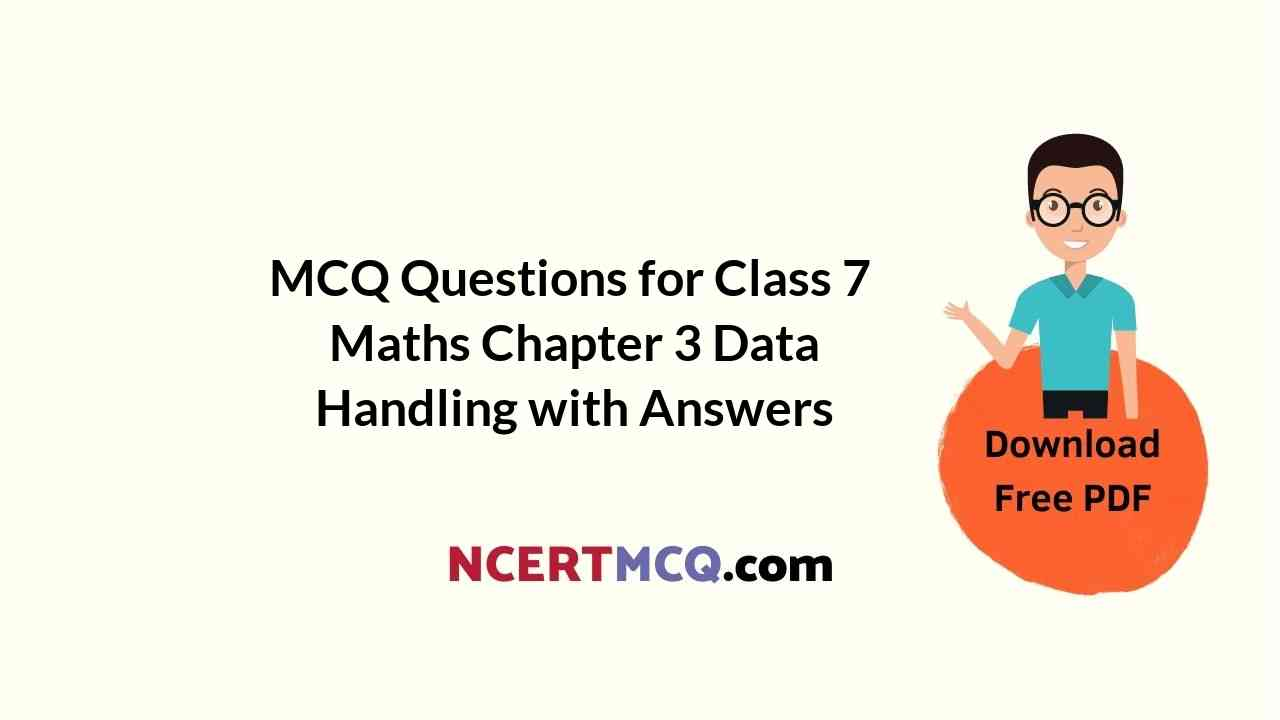Check the below NCERT MCQ Questions for Class 7 Maths Chapter 3 Data Handling with Answers Pdf free download. MCQ Questions for Class 7 Maths with Answers were prepared based on the latest exam pattern. We have provided Data Handling Class 7 Maths MCQs Questions with Answers to help students understand the concept very well. https://ncertmcq.com/mcq-questions-for-class-7-maths-with-answers/

Students can also refer to NCERT Solutions for Class 7 Maths Chapter 3 Data Handling for better exam preparation and score more marks.

## Data Handling Class 7 MCQs Questions with Answers

The number of tourists visiting a historical place in a week is shown in the following table :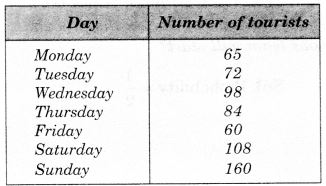Data Handling Class 7 MCQ Question 1.
On which day is the number of tourists maximum?
(a) Sunday
(b) Wednesday
(c) Tuesday
(d) Saturday

Hint:
Sunday → 160.

Class 7 Maths Chapter 3 MCQ Question 2.
On which day is the number of tourists minimum?
(a) Friday
(b) Monday
(c) Thursday
(d) Saturday

Hint:
Friday → 60.

MCQ On Data Handling For Class 7 Question 3.
On which day 60 tourists visit?
(a) Monday
(b) Tuesday
(c) Friday
(d) Sunday

Hint:
60 → Friday.

MCQ Questions For Class 7 Maths Chapter 3 Question 4.
What is the difference between the number of tourists visiting on Friday and Monday?
(a) 10
(b) 24
(c) 38
(d) 5

Hint:
65 – 60 = 5.

Class 7 Maths Chapter 3 MCQ With Answers Question 5.
The sum of the number of tourists visiting on Sunday and Friday is
(a) 160
(b) 60
(c) 220
(d) 100

Hint:
160 + 60 = 220.

Data Handling MCQ Class 7 Question 6.
The difference between the maximum and minimum number of tourists is
(a) 50
(b) 80
(c) 90
(d) 100

Hint:
160 – 60 = 100.

Class 7 Math Chapter 3 MCQ Question 7.
A batsman scored the following number of runs in six innings:
35, 30, 45, 65, 39, 20
The mean runs scored by him in an inning is
(a) 39
(b) 38
(c) 37
(d) 40

Hint:
Mean = $$\frac { 35+30+45+65+39+20 }{ 6 }$$
= $$\frac { 234 }{ 6 }$$ = 39

Class 7 Maths Ch 3 MCQ Question 8.
The mean of the numbers 10,20, 30 and 40 is
(a) 20
(b) 25
(c) 30
(d) 50

Hint:
Mean = $$\frac { 10+20+30+40 }{ 4 }$$ = $$\frac { 100 }{ 4 }$$ = 25.

MCQ Class 7 Maths Chapter 3 Question 9.
The range of the weights (in kg) of a students of a class given below is:
49, 60, 47, 50, 47, 59, 58, 45, 53
(a) 10
(b) 15
(c) 20
(d) 2

Hint:
Range = 60 – 45 = 15.

Class 7 Data Handling MCQ Question 10.
The marks of 11 students of a class are as given below:
78, 11, 99, 63, 94, 6, 78, 36, 30, 55, 22
The range of marks is
(a) 90
(b) 91
(c) 92
(d) 93

Hint:
Range = 99 – 6 = 93.

Ncert Class 7 Maths Chapter 3 MCQ Question 11.
The rainfall (in mm) in a city on 7 days of a certain week was recorded as follows: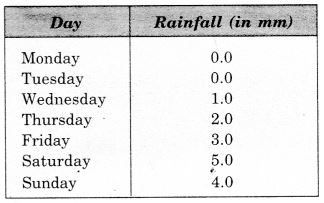On how many days was the rainfall less than 6 mm?
(a) 0
(b) 3
(c) 6
(d) 7

Hint:
On each day,rainfall < 6 mm.

Class 7th Maths Chapter 3 MCQ Question 12.
The mode of the distribution 3,5, 7, 4, 2, 1, 4, 3, 4 is
(a) 7
(b) 4
(c) 3
(d) 1

Hint:
4 occurs maximum number (3) times.

MCQ Questions For Class 7 Maths Data Handling Question 13.
The marks of some students are as given below:
30, 31, 32, 32, 33, 32, 34, 35, 30, 31, 33, 32
Find the mode of their marks.
(a) 30
(b) 31
(c) 32
(d) 33

Hint:
32 occurs maximum number (4) times.

Class 7 Chapter 3 Maths MCQ Question 14.
The median of the distribution 2, 3, 4, 7, 5, 1, 6 is
(a) 1
(b) 2
(c) 3
(d) 4

Hint:
1, 2, 3, 4, 5, 6, 7.

Class 7 Maths MCQ Chapter 3 Question 15.
The median of the data 20, 30, 40, 10, 15, 25, 35 is
(a) 20
(b) 25
(c) 30
(d) 40

Hint:
10, 15, 20, 25, 30, 35, 40.

Question 16.
Which of the following statements is true?
(a) The mode is always one of the numbers in a data
(b) The mean is always one of the numbers in a data
(c) Mean < Mode in a data
(d) Median < Mode in a data

Answer: (a) The mode is always one of the numbers in a data

Question 17.
A coin is tossed. What is the probability of getting head?
(a) 0
(b) 1
(c) $$\frac { 1 }{ 2 }$$
(d) 2

Answer: (c) $$\frac { 1 }{ 2 }$$
Hint:
A coin has two faces.

Question 18.
A coin is tossed. What is the probability of getting tail?
(a) 1
(b) $$\frac { 1 }{ 2 }$$
(c) 2
(d) 0

Answer: (b) $$\frac { 1 }{ 2 }$$
Hint:
A coin has two faces.

Question 19.
A die is thrown. What is the probability of getting 1?
(a) 0
(b) 1
(c) $$\frac { 1 }{ 2 }$$
(d) $$\frac { 1 }{ 6 }$$

Answer: (d) $$\frac { 1 }{ 6 }$$
Hint:
A die has six faces.

Question 20.
A die is thrown. What is the probability of getting 6?
(a) 0
(b) $$\frac { 1 }{ 6 }$$
(c) $$\frac { 1 }{ 2 }$$
(d) 1

Answer: (b) $$\frac { 1 }{ 6 }$$
Hint:
A die has six faces.

Read the following bar graph and answer the following related questions :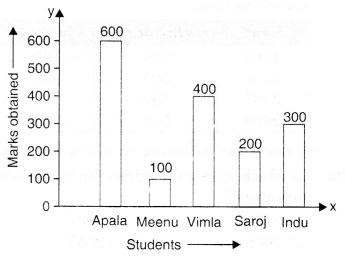Question 21.
Who got the maximum marks?
(a) Apala
(b) Meenu
(c) Saroj
(d) Indu

Hint:
Apala → 600.

Question 22.
Who got the minimum marks?
(a) Apala
(b) Meenu
(c) Vimla
(d) Indu

Hint:
Meenu → 100.

Question 23.
The difference between maximum and minimum marks is
(a) 100
(d) 200
(c) 400
(d) 500

Hint:
600 – 100 = 500.

Question 24.
The ratio between the marks obtained by Saroj and Vimla is
(a) 1 : 2
(b) 2 : 3
(c) 3 : 4
(d) 1 : 6

Hint:
200 : 400 = 1 : 2.

Question 25.
How many girls have got marks more than 100?
(a) 2
(b) 3
(c) 4
(d) 1

Hint:
200, 300, 400, 500 → 4.

Question 26.
Who has got 400 marks?
(a) Vimla
(b) Saroj
(c) Indu
(d) Apala

Hint:
400 → Vimla.

Question 27.
The difference between the marks obtained by Vimla and Saroj is how many times the difference between the marks obtained by Meenu and Saroj?
(a) 2
(b) 3
(c) 4
(d) 6

Hint:
400 – 200 = 200; 200 – 100 = 100; 200 = 2 x 100.

Question 28.
How many girls have got marks less than 600?
(a) 1
(b) 2
(c) 3
(d) 4

Hint:
100, 200, 300, 400 → 4.

Sale of Mathematics and English books in the year 2005, 2006, 2007 and 2008 are given below :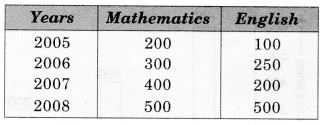Answer the following related questions :

Question 29.
In which year is the difference in the sale minimum?
(a) 2008
(b) 2007
(c) 2006
(d) 2005

Hint:
2008 → 500 – 500 = 0.

Question 30.
In which year is the difference in the sale maximum?
(a)2005
(b) 2006
(c) 2007
(d) 2008

Hint:
400 – 200 = 200.

Question 31.
The ratio of sales in the year 2005 is
(a) 2 : 1
(b) 3 : 1
(c) 4 : 1
(d) 2 : 3

Hint:
200 : 100 = 2 : 1.

Question 32.
The rise in the sale of Mathematics books from 2005 to 2008 is
(a) 100
(b) 200
(c) 300
(d) 400

Hint:
500 – 200 = 300.

Question 33.
The fall in the sale of English books from 2006 to 2007 is
(a) 50
(b) 100
(c) 150
(d) 200

Hint:
250 – 200 = 50.

Number of children in six different classes are given below :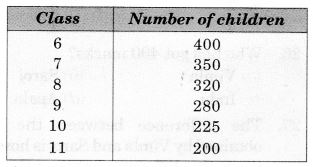Question 34.
In which class is the number of children maximum?
(a) 6
(b) 7
(c) 8
(d) 9

Hint:
6 → 400.

Question 35.
In which class is the number of children minimum?
(a) 8
(b) 9
(c) 10
(d) 11

Hint:
11 → 200.

Question 36.
The difference between the maximum and minimum number of children is
(a) 100
(b) 200
(c) 300
(d) 400

Hint:
400 – 200 = 200.

Question 37.
In how many classes is the number of children less than 500?
(a) 2
(b) 4
(c) 5
(d) 6

Hint:
In each class, number of students < 500.

Question 38.
In how many classes is the number of children more than 100?
(a) 6
(b) 4
(c) 3
(d) 1

Hint:
In each class, number of students > 100.

Question 39.
The ratio of the number of children of class 6 and 11 is
(a) 3 : 1
(b) 2 : 1
(c) 2 : 3
(d) 1 : 4

Hint:
400 : 200 = 2 : 1.

Question 40.
The total number of children is
(a) 1775
(b) 1675
(c) 1575
(d) 1785